Click Question to Get Free Answers >

# Conic Sections NCERT Solutions : Class 11 Maths

## NCERT Solutions for Class 11 Maths : Conic SectionsFilter
Filters :
Classes
•
•
•
•
•
•
•
Chapters
•
•
•
•
•
•
•
•
•
•
• 6 More
Exercises
•
•
•
•
•
•

Doubtnut one of the best online education platform provides free NCERT Solutions of Maths for Class 11 Conic Sections which are solved by our maths experts as per the NCERT (CBSE) guidelines. We provide the solutions as video solutions in which concepts are also explained along with answer which will help students while learning , preparing for exams and in doing homework. These Solutions will help to revise complete syllabus and score more marks in examinations.

Get here free, accurate and comprehensive NCERT Solutions for Class 11 Maths Conic Sections which have been reviewed by our maths counsellors as per the latest edition following up the CBSE guidelines. We provide video solutions in which solutions to all the questions of NCERT Class 11 Maths textbook are explained in step by step and detailed way.

## The Topics of the Chapter Conic Sections are :

STANDARD EQUATION OF PARABOLA

INTRODUCTION

EQUATION OF THE ELLIPSE IN STANDARD FORM

EQUATION OF TANGENT

APPLICATIONS OF PARABOLA

INTERSECTION OF A LINE AND A PARABOLA

GENERAL EQUATION OF CIRCLE

CONJUGATE HYPERBOLA

POSITION OF POINTS WITH RESPECT TO A CIRCLE

DIFFERENT CASES FOR INTERSECTION OF A LINE AND A CIRCLE

EQUATION OF CIRCLE WITH TYPICAL CONDITIONS

It contains these exercises along with solved examples. All the exercises are solved in the video. Select the exercise to view the solutions exercisewise of the Chapter Conic Sections:

## NCERT Solutions Class 11 Maths Chapter Conic Sections Exercises:

We have covered all the exercises and also Solved examples in the videos. Along with the practise exercise Students should also practise solved examples to clear the concepts of the Conic Sections. If incase you have any doubt you can watch the solutions for the given questions.Watch the solutions for the given questions in which it is also explained in the video steps to solve the questions along with answers.

In this chapter, we will focus on some particular curves namely circles, ellipses, parabolas and hyperbolas. It will elucidate the intersection of a plane with a double-napped right circular cone resulting in different types of curves. It would also be possible to get information on other topics such as latus rectum as well. Doubtnut has made it possible to clear the queries of the students by providing the right idea on how to attempt the different questions without any problem at all. It boosts the confidence of the students where they can always expect to score good marks in the examination.

CBSE curriculum follows NCERT textbooks. Thus, NCERT textbooks are essential because, in some instances, the question is asked directly from the NCERT textbook in the CBSE exams. When students appear for their Maths examination, they find specific questions quite confusing. It is because they are unable to clear their queries on particular topics that lead to frustration and poor marks in academics. NCERT Math class 11 textbook helps in explaining the basic concepts in simple and lucid language, but it lacks enough solved examples for the in-depth understanding of the complex mathematical concepts. So, in this case, students look forward to the different guide books which consume lot of time and money. For subjects like Maths, if the concepts are not understood properly, then it would be impossible to be successful in the exams. So, it all needs the right amount of practice and effective learning approach to scoring high marks in the subject.

To impart the best and accurate solutions to the students’ doubts, a team of expert tutors at Doubtnut have prepared video tutorials for  maths NCERT solutions. With the help of the step by step instructions by the expert tutors, students do not find it difficult at all to get their queries cleared. So, the right decision taken by the students to opt for the online study materials can genuinely help to serve your exact purpose. It would help to clear all their queries where it would never confuse them in their exams. Also the Class 11 Maths NCERT Solutions are provided in pdf format that can be downloaded and accessed anywhere and anytime. Moreover, the NCERT solutions provided by Doubtnut are helpful in quick revision for preparation of competitive exams like IIT JEE Mains and Advanced.

## Topics included NCERT Class 11 Maths Solutions Chapter 11 Conic Sections

### Introduction

In this chapter, we will focus on different types of curves. Concepts such as hyperbola and parabola were given by Apollonius. These are known as conic sections. Well, let’s proceed further to have a clear idea about it in the best way.

### Sections of a Cone

Let there be a fixed vertical line, ‘l’ along with another line, ‘m’ intersecting at a fixed point, ‘V.’ It is inclined at an angle α.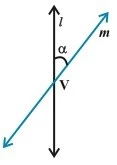Taking into consideration that the angle α remains constant, if we rotate the line, ‘m’ around the line, ‘l’, there would be a generation of services which would be a double-napped right circular hollow cone.  This is known as cone (Fig 11.2)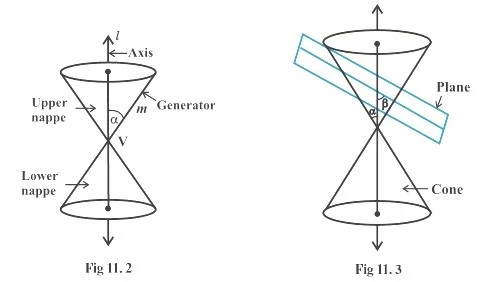The ‘l’ happens to be the axis of the cone while the point V is called the vertex. You can find a rotating line ‘m.’ This is called a generator of the cone. Here, the cones are separated by the vertex into two parts, which are called nappes. By taking the intersection of a plane with a cone, the obtained section is known as a conic section. Let β be the angle made by the intersecting plane with the vertical axis of the cone (Fig 11.3).

### Circle, ellipse, parabola, and hyperbola

When the nappe is cut by the plane of the cone, we get the following situations.

1. In Fig 11.4, the section is a circle when β = 90o
2. In Fig 11.5, the section is an eclipse when α < β <  90o

(c) In Fig 11.6, the section is a parabola when β = α

(d) In Fig 11.7, the section is a hyperbola when 0 ≤ β < α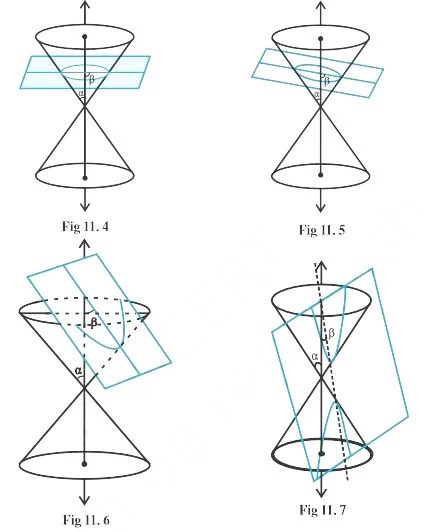### Degenerated conic sections

When the vertex of the cone is cut by the plane, we get the following cases:

1. The section is a point when  α < β ≤ 90o (Fig 11.8)
2. The section is a straight line when  β = α (Fig 11.9) (parabola)
3. The section is a pair of intersecting straight lines when  0 ≤ β < α (Fig 11.9) (hyperbola)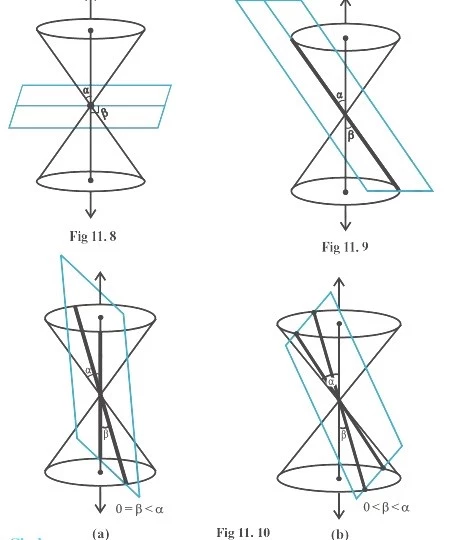### Circle

Here, we will learn about the equation of the circle.  Look at the Fig 11.11 where you can find the radius of the circle.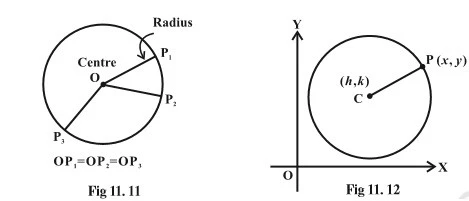If the center of the circle is at the origin, then the equation of the circle is simplest. In Fig 11.12 with a given radius and center, we derive below the equation of the circle.

Given C (h, k) be the center and r the radius of circle. Let P(x, y) be any point on the circle (Fig11.12).  Then, by the definition, | CP | = r. By the distance formula, we have: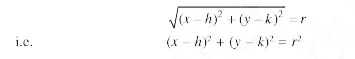Example:  Find the centre and the radius of the circle x2 + y2 + 8x + 10y –     8 = 0

Solution: The equation given is (x2 + 8x) + (y2 + 10y) = 8

By making a completion of the squares within the parenthesis, we get:

(x2 + 8x + 16) + (y2 + 10y + 25) = 8 + 16 + 25

i.e. (x + 4)2 + (y + 5)2 = 49

i.e. {x – (– 4)}2 + {y – (–5)}2 = 72

So, it can be concluded that the given circle has the center at (-4, -5) and radius 7.

Parabola

Para’ means ‘for’ and ‘bola’ means ‘throwing.’ The set of all points in a plane which are equidistant from both fixed point and the fixed line is called a parabola.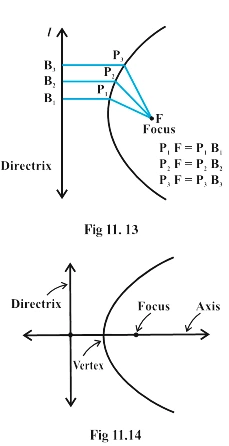In Fig 11.13, the directrix of the parabola is the fixed line. On the other hand, the fixed point F is known as the focus.

In Fig 11.14, the axis of the parabola is the line which is seen through the focus and perpendicular to the directrix.

### Latus rectum

When a line segment is perpendicular to the axis of the parabola through the focus, then this is known as Latus rectum of a parabola. Look at the Fig 11.17 where the end points lie on the parabola.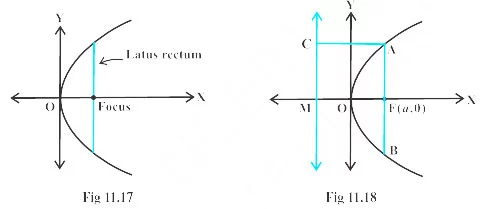Let’s find the length of the latus rectum of the parabola y2 = 4ax (Fig 11.18)

As we know that the definition of the parabola, so we will apply here,

AF = AC

But            AC = FM = 2a

Hence        AF = 2a

And since the parabola is symmetric with respect to x-axis AF = FB and so

AB = Length of the latus rectum = 4a.

### Ellipse

An ellipse happens to be the set of all points in a plane. Here the sum of the two distances from two fixed points in the plane is a constant. The foci is the two points. It is to be noted that the centre of the eclipse is the midpoint of the line segment joining the foci.  Look at the figure below: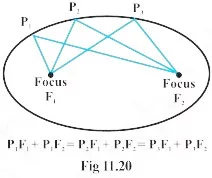In Fig 11.21, the vertices of the ellipse are the end points of the major axis. The length of the major axis is denoted by 2a, whereas 2b is the length of the minor axis. Similarly a and b are the length of the semi-major axis and semi-minor axis respectively (Fig 11.22)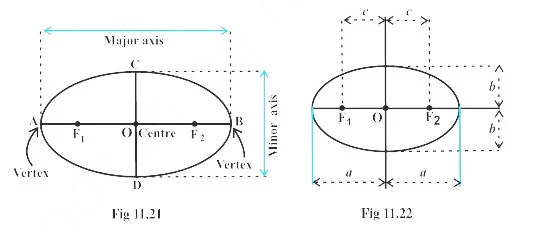### Hyperbola

The set of all points in a plane where the difference are seen from two fixed points in the plane is constant is known as a hyperbola.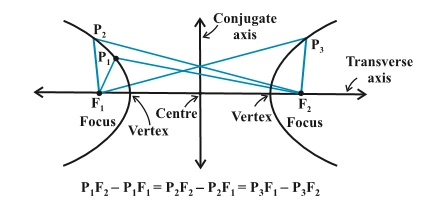Here in this figure, you can find that there are two fixed points which is known as foci of the hyperbola. The transverse axis is the line through the foci and the conjugate axis is the line which is seen through the centre and perpendicular to the transverse axis.

The distance between the two foci is denoted by 2c where 2a is denoted as the distance between two vertices.  We define the quantity b as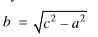It is to be mentioned that 2b happens to be the length of the conjugate axis (Fig 11.30)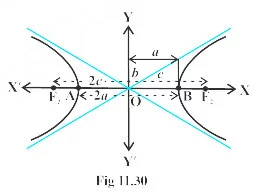In order to find the constant P1F2 – P1F1 :

By taking the point P at A and B   in the Fig 11.30, we have

BF1 – BF2 =  AF2 – AF1 (by the definition of the hyperbola)

BA +AF1– BF2 = AB + BF2– AF1

i.e., AF1 = BF2

So that, BF1 – BF2 =  BA + AF1– BF2 = BA = 2a

### Standard equation of closest

If the center of the hyperbola is at the origin and also the foci are on the x-axis or y-axis, then the equation of the hyperbola is simplest. There can be two such possible orientations. Let’s have a look at the Fig 11.31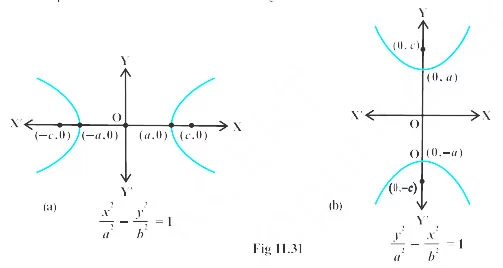Let us assume the foci to be F1 and F2 and be the midpoint of the line segment F1F2.  Here let F2 be the positive x-axis and the negative x-axis be the F1 Here O is the origin. Let the coordinates of F1 be (– c,0) and F2 be (c,0) (Fig 11.32).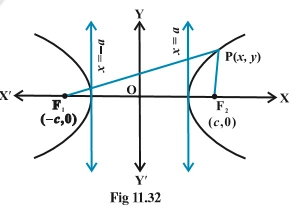Here, let P (x, y) be any point on the hyperbola and the closer point be 2a.

So given, PF1 – PF2 = 2a

With the use of distance formula, we have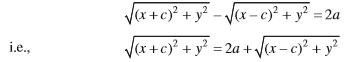Squaring both sides, we get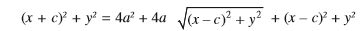and on simplifying, we get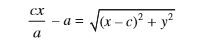On squaring again and further simplifying, we get

x-    y2      =1

a2       c2 – a2

i.e.        x-    y2   =1              (Since c2 – a2 = b2)

a2           b2

Hence, it is seen that any point on the hyperbola satisfies

x-    y2    =1

a2         b2

Conversely, let P(x, y) satisfy the above equation with 0 < a < c. Then

Therefore,     PF1

= a + c/a  x

Similarly,    PF2 = a - a /c x

In hyperbola c > a; and since P is to the right of the line x = a, x> c /ax > a

Therefore   a- c/a x becomes negative

Thus PF2 = c/a x - a

Therefore, PF1 – PF2 = a + c x/a – cx /a+ a =2a

It can be noted that if P is to the left of the line x = -a, then

PF1 = - (a + c/a x), PF2 = a - c/a x

So, PF2 – PF1 = 2a

It should be kept in mind that any point that satisfies x2/a2 – y2/b2 = 1, always lie on the

hyperbola.

Therefore, it has been proved that the equation of hyperbola with origin (0,0) and transverse axis along x-axis is       x2 y2 = 1

a2   b2

So, in Fig 11.31(b) we can derive the equation of the hyperbola as

y2/a2 – x2/b2 = 1

These two equations are known as the standard equations of hyperbolas.

## Significance of Doubtnut for Class 11 Maths students

The online study materials prepared by the experts of Doubtnut can help a lot to impart the right education to the students. They begin to fall in love with the subject they hated the most. So, Doubtnut has really played a significant role by imparting the best form of online education. With the inclusion of NCERT Class 11 Maths Solutions Chapter 11, it has proved to be quite advantageous for students to prepare for their academic examinations and board examinations. Let us have a look at the different benefits of learning math from

• Quite easy to understand with examples: The online study materials are quite easy to understand. It is because it has been prepared using the most straightforward language so that the students do not get confused at all. This indeed helps a lot to find the perfect idea on how to attempt various questions correctly. So, scoring good marks become quite possible where they do not have to get disappointed at all.
• Saves both time and money: By making the right decision to follow the most useful online video tutorials, it can truly help to save both money and time.  The reason behind this is the fact that it is free of cost where one does not have to pay a single penny for it. Therefore, it can be possible to get the maximum advantage out of it.
• Ease of Access: NCERT solutions can be downloaded in the pdf format where students can access it without any problem at all. Thus, students can prepare for their exams and keep themselves abreast of their syllabus. Thus the right decision to follow the best online study materials can genuinely help to pass the exams with flying colors.